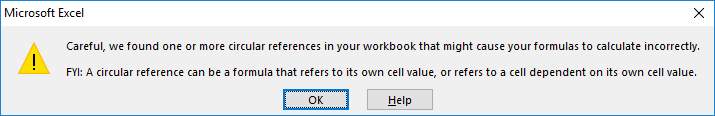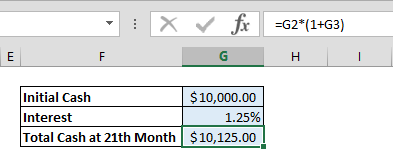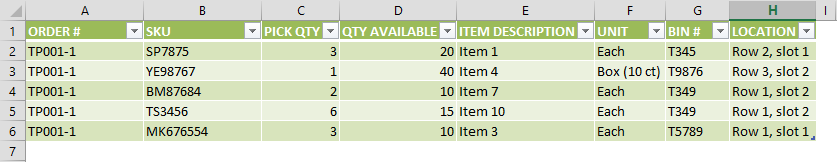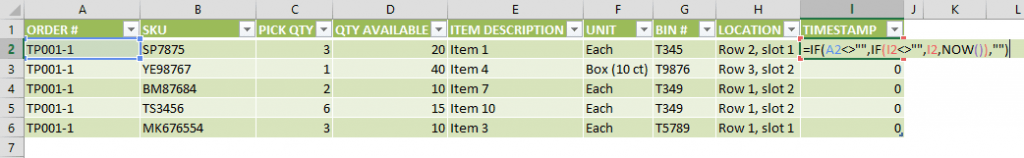Iterative calculations can help find the solution to mathematical problems by running calculations over and over using previous results. This is made possible by computers that can run calculations repeatedly to find the likelihood of possible answers by getting closer to the results from different angles.

In Excel, you can reference a cell that contains a formula and use its result in an identical formula in a different cell. For this, you would need to copy the formula and references as many times as you want to repeat the process. This can work if your model is relatively simple, but doing so in more complicated workbooks might prove much more challenging, if not downright impossible to do.

An alternative and better approach is to use the Excel iterative calculation feature. You can create a formula that refers to the cell containing the formula. The formula can use the result of the previous calculations, thus automatically calculating the same thing over multiple iterations.

As easy as it sounds, there are a few things you need to consider. First of all, the number of iterations should be limited. Even though a higher iteration count usually means more accurate results, this also means longer calculations times – and sometimes crashes. Another thing to note is that when iterative calculations are disabled, Excel will show a warning as circular references are usually considered user errors unless you know what you’re doing.# Enabling Excel Iterative Calculations

To activate and use circular references, you must first activate them by checking Enable iterative calculations option under the File menu.

Go to File > Options > Formulas > Calculation options section in Excel 2016, Excel 2013 and Excel 2010.In Excel 2007, go to Office button > Excel options > Formulas > Iteration area.

In Excel 2003 and earlier, go to Menu > Tools > Options > Calculation.

Enabling iterative calculations will bring up two additional inputs in the same menu:

• Maximum Iterations determines how many times Excel is to recalculate the workbook,
• Maximum Change determines the maximum difference between values of iterative formulas. Note that entering a smaller number here means more accurate results.

Iterative calculations stop when one of the conditions defined (iterations count or change value) are matched. For example, let’s assume that Maximum Iterations is set to 100 and Maximum Change to 0.001. This means that Excel will stop calculating either after 100 calculations, or when there’s less than 0.001 difference between the results.

# Use Cases

## Calculating Future Value of an Investment

Let’s assume that we have \$10,000 and want to invest this money in a cash deposit (CD) account. We’re going to assume a monthly interest rate of 1.25%. You can download the sample workbook for this use case . To calculate the total value at the end of the 21st month, we’re going to calculate the principal for each month, and add the interest to the previous month.Begin by entering the starting cash, interest, and the total value function like below.

=value * ( 1 + interest rate)Then, select the cell with the initial cash value and add the reference of the total value function.This will give a circular reference warning if iterative calculations are not enabled. If you haven’t done so already, enable this option and set the Maximum Iterations to 20 to find the interest for the 21st month. See the previous section Enabling Iterative Calculations to enable this feature.## Automatic Timestamp

Circular references can also be used to add time stamps into cells. You can download the sample workbook for this use case . Let’s assume we want to add time stamps to the orders entered in the table below.We can use circular references to add a time stamp when a new order information is entered. To do this, begin by adding a new column into the table where you’d like to print the timestamps. Type in the formula,

=IF(A2<>””,IF(I2<>””,I2,NOW()),””)

This formula will check whether there’s data in Order Number (cell A2). If it’s not blank and the timestamp cell is empty, the formula will return the NOW() function.Note that pressing the Enter key will give a circular reference warning if iterative calculations are not enabled. See the previous section Enabling Iterative Calculations to enable this feature. This time Maximum Iterations or Maximum Change numbers don’t mean much, because we only need a single iteration, so you can leave these two inputs in their default values.Now, every time we enter a new order and create a new row, a timestamp will be automatically printed on the Timestamp column.